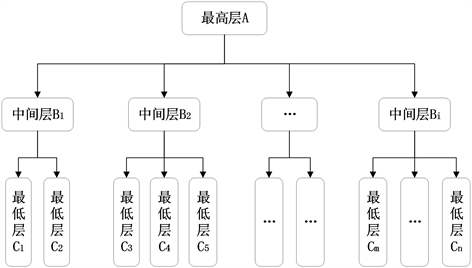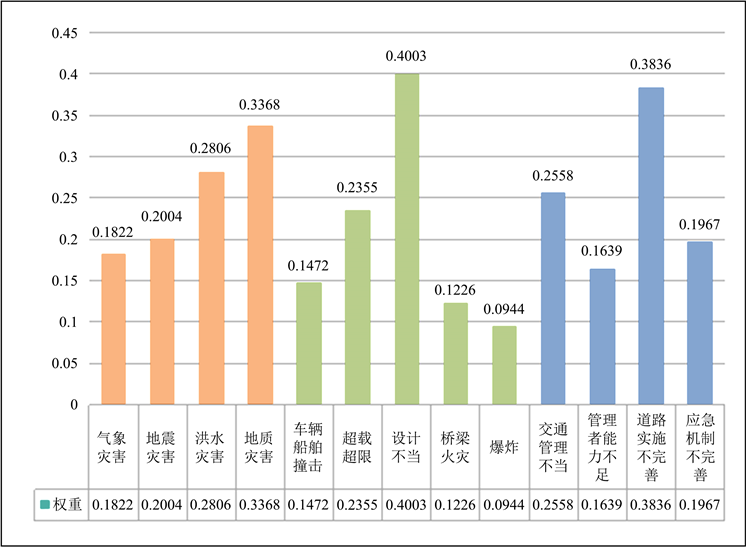#### 期刊菜单

Application of IAHP in Risk Assessment of Bridge Operation
DOI: 10.12677/HJCE.2023.125061, PDF , HTML, XML, 下载: 61  浏览: 105

Abstract: To ensure the safety and reliability of bridge engineering, it is necessary to evaluate the operational risks of bridge engineering. This article proposes a method for evaluating operational risks of bridge engineering using the Improved Analytic Hierarchy Process (IAHP). Based on the traditional AHP, the scaling method is used to construct the judgment matrix, and the transitivity between indicators is used to avoid consistency testing of the traditional method, simplifying the calculation. This method can effectively identify the main factors from various levels of risk indicators, facilitating the quantification of risk assessment indicators during the operational phase of bridge engineering. It can provide scientific and reasonable reference for bridge managers and maintainers, and improve the operational risk management level of bridge engineering.

1. 引言

2. 层次分析法

2.1. 层次分析法的局限性Table 1. Meaning of common scale values

$R=\left[\begin{array}{ccc}1& 9& 9\\ \text{1/9}& 1& 9\\ \text{1/9}& \text{1/9}& 1\end{array}\right]$

${R}^{\prime }=\left[\begin{array}{ccc}1& 1.8& 1.8\\ \text{1/1}\text{.8}& 1& 1.8\\ \text{1/1}\text{.8}& \text{1/1}\text{.8}& 1\end{array}\right]$

2.2. 标度扩展法构造判断矩阵

$R=\left[\begin{array}{ccccc}1& {t}_{1}& {t}_{1}{t}_{2}& \cdots & \underset{i=1}{\overset{n-1}{\prod }}{t}_{i}\\ 1/{t}_{1}& 1& {t}_{2}& \cdots & \underset{i=2}{\overset{n-1}{\prod }}{t}_{i}\\ 1/{t}_{1}{t}_{2}& 1/{t}_{2}& 1& \cdots & \underset{i=3}{\overset{n-1}{\prod }}{t}_{i}\\ ⋮& ⋮& ⋮& \ddots & ⋮\\ 1/\underset{i=1}{\overset{n-1}{\prod }}{t}_{i}& 1/\underset{i=2}{\overset{n-1}{\prod }}{t}_{i}& 1/\underset{i=3}{\overset{n-1}{\prod }}{t}_{i}& \cdots & 1\end{array}\right]$

$\text{CR}=\frac{\text{CI}}{\text{RI}}=\frac{{\lambda }_{\mathrm{max}}-n}{\left(n-1\right)\text{RI}}$ (1)

2.3. 标度扩展法构造判断矩阵Figure 1. The hierarchical model of AHPTable 3. Judgment matrix A-B of the first level indicator layerTable 4. Judgment matrix Bi-C for the second level indicator layer

${\alpha }_{i}=\sqrt[n]{\underset{j=1}{\overset{n}{\prod }}{r}_{ij}}/\underset{i=1}{\overset{n}{\sum }}\sqrt[n]{\underset{j=1}{\overset{n}{\prod }}{r}_{ij}}$

3. RM桥梁工程运营风险评估

3.1. 构建风险评估指标体系Figure 2. Risk assessment index system for RM bridges

3.2. 评价指标权重计算Table 5. Comparison table of risk management factors for bridge operations

${R}_{A}=\left[\begin{array}{ccc}1& 0.566& 0.463\\ 1.8& 1& 0.833\\ 2.16& 1.2& 1\end{array}\right]$Figure 3. Comparison of weight values of RM bridge risk assessment indicators

4. 结语

  陈红, 陈治, 金珠, 张效红, 周立燕. 基于层次分析和灰色关联法的桥梁运营阶段风险评价[J]. 筑路机械与施工机械化, 2019, 36(8): 112-115.  吴伟东, 姚灿, 潘海泽, 许博浩, 黄鹏. 装配式建筑项目实施阶段质量风险评估研究[J]. 科技促进发展, 2020, 16(10): 1259-1265.  张荣凤, 亓兴军. 运营期桥梁风险评估综述[J]. 中国水运(下半月), 2020, 20(10): 123-125.  Tempa, K. (2022) District Flood Vulnerability Assessment Using Analytic Hierarchy Process (AHP) with Historical Flood Events in Bhutan. PLOS ONE, 17, e0270467. https://doi.org/10.1371/journal.pone.0270467  Karimi, S. and Mirza, O. (2023) Damage Identification in Bridge Structures: Review of Available Methods and Case Studies. Australian Journal of Structural Engineering, 24, 89-119. https://doi.org/10.1080/13287982.2022.2120239  赵书强, 汤善发. 基于改进层次分析法、CRITIC法与逼近理想解排序法的输电网规划方案综合评价[J]. 电力自动化设备, 2019, 39(3): 143-148.  段洪琳, 邹晓芳, 杨德元, 李美京, 陈兵硕, 李佳. 桥梁运营安全风险源辨识及预警指标体系研究——以鄂东长江公路大桥为例[J]. 交通工程, 2021, 21(1): 80-85.  甄逸群. 工程建设项目风险应对策略[J]. 水利水电技术(中英文), 2022, 53(S2): 69-71.  母荣华. 云南省WM高速公路工程项目运营管理阶段风险控制研究[D]: [硕士学位论文]. 成都: 西南交通大学, 2020.  杨树志. 海河斜拉桥运营风险评估[D]: [硕士学位论文]. 兰州: 兰州交通大学, 2019.  刘健锋, 韦勇克, 刘远锋. 基于安全设防等级划分与模糊层次分析的桥梁运营安全评价[J]. 公路交通科技, 2020, 37(10): 107-117.  曹廷, 章世祥, 朱森林. 江苏省在役桥梁运营期安全风险多域源递进综合评价[J]. 工程技术研究, 2021, 6(19): 28-30.  刘琴. 山区高速公路桥梁营运期的安全风险评估[J]. 公路交通科技(应用技术版), 2020, 16(6): 321-324.  罗郑. JF公司桥梁工程风险管理研究[D]: [硕士学位论文]. 成都: 电子科技大学, 2016.  陈治. 在役梁桥的风险现状分析及安全评价与风险控制研究[D]: [硕士学位论文]. 昆明: 昆明理工大学, 2017.